# 3.5 Polygons and Shapes

## 3.5 Polygons and Shapes

When lines meet and form complete boundaries, they make shapes with different numbers of sides. These shapes are called polygons. The polygon with the fewest number of sides is a triangle. It has three sides and three angles. A quadrilateral is bounded by four sides. There is no limit to the number of sides a polygon may have.

To summarize then, a polygon is a shape formed by the intersection of three or more lines that make a boundary. The points where the lines meet are called vertices; these are the vertices of the polygon. At these vertices, angles are formed. The lines themselves form the edges of the polygon.

• A regular polygon is one whose sides and angles are equal.

• Congruent shapes are any two shapes whose angles are equal and sides are proportional (in the same ratio).

• A simple polygon has no internal edges, and therefore has no edges that intersect themselves. Figures 3.8 and 3.10 show simple polygons.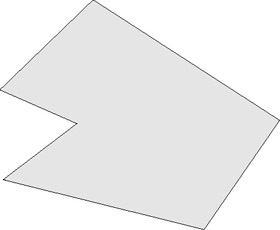Figure 3.8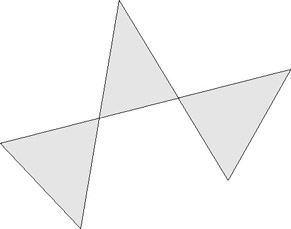Figure 3.9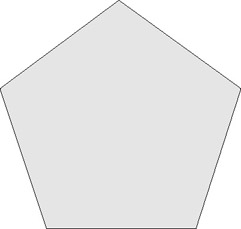Figure 3.10

• A complex polygon has internal edges and intersects itself, as in Figure 3.9.

• A convex polygon is a simple polygon whose angles are all less than reflex angles. If a polygon is not convex, it is said to be concave.

### 3.5.1 Triangles

A triangle is the simplest polygon. It is made up of three sides and has three angles. Using a combination of triangles, every other polygon can be formed. For example, squares are made up of two adjacent triangles. The sum of angles in a triangle is equal to two right angles, and at least two angles in every triangle are acute. There are four types of triangles.

• Equilateral triangle-All sides and angles are equal.

• Isosceles triangle-Has at least two equal sides and angles.

For this reason, the equilateral triangle is also a form of the isosceles triangle.

• Scalene triangle-No sides or angles are equal.

• Right triangle-Has one angle that is a right angle, and so the other two angles are complementary angles.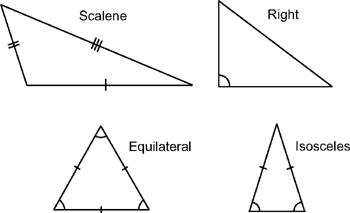Figure 3.11: Reproduced from Wikipedia under the GNU license

 Note When faced with a simple polygon that has any number of sides, you can determine how many triangles it is composed of by subtracting 2 from the number of sides. The result will be the number of triangles. For example, how many triangles are in a square? A square has four sides, so 4 − 2 = 2, which means two triangles.

### 3.5.2 Squares

A square is composed of two right triangles. We know the total angle sum in a triangle is two right angles, and therefore the total sum in a square must be twice this amount. A square has four equal sides and four right angles.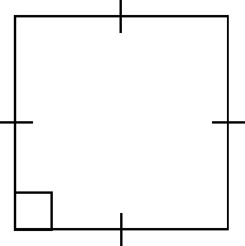Figure 3.12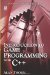Introduction to Game Programming with C++ (Wordware Game Developers Library)
ISBN: 1598220322
EAN: 2147483647
Year: 2007
Pages: 225
Authors: Alan Thorn

Similar book on Amazon

flylib.com © 2008-2017.
If you may any questions please contact us: flylib@qtcs.net